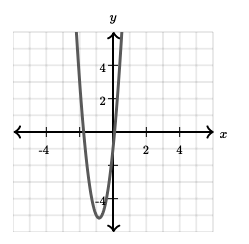Know more about the Repeaters Batch Know more about the Repeaters Batch

# Condition for common roots

An algebraic polynomial can be defined as the algebraic expression that involves many terms of the form cxn, where n is non-negative in nature. For example: 3x3 – 2x2 + 5x – 6. A polynomial with its degree 2 can be termed a quadratic polynomial. If f (x) is said to be a quadratic polynomial, then f (x) being equated to 0 is a quadratic equation. The solution of a quadratic equation can be found using various methods such as factoring, square root, completing the square, quadratic formula, graph of a quadratic polynomial. The quadratic formula method is most commonly used. The standard representation of a quadratic equation is ax2 + bx + c = 0, where a ≠ 0. The values a, b and c denote real numbers. If a becomes 0, then the equation will reduce to bx + c = 0, which is a linear equation, and not a quadratic equation. The value of the discriminant determines the type of solution or roots. Discriminant can be found using the formula: D = b2 – 4ac. There exist 3 different cases:

1] When D > 0: real and distinct roots.

2] When D = 0: real and equal roots.

3] When D < 0: imaginary and roots in pairs.

Illustration: Solve the quadratic equation 6x2 + 10x – 1 = 0.

From the above problem, a = 6, b = 10, c = – 1.

To find the value of determinant: D = b2 – 4ac is used

D = (10)2 – 4 * 6 * (- 1)

= 100 + 24

= 124

D = 124

D > 0

Therefore, from the value of the determinant, we can conclude that the roots are real and distinct.

Using the quadratic formula: x = [- b ± √b2 – 4ac] / 2a

= [- 10 ± √102 – 4 * 6 * (- 1)] / (2 * 6)

= [- 10 ± √124] / 12

The two roots are x = [- 10 – √124] / 12 and [- 10 + √124] / 12.

The graph of the quadratic equation is as follows:The roots of the quadratic equation are given by x = (- b + √discriminant) / 2a and x = (- b – √discriminant) / 2a.

Let these roots be ɑ and β.

The sum of the roots ɑ and β is given by

α + β = (- b + √discriminant) / 2a + (-b – √discriminant) / 2a

= (- b / 2a ) + (√discriminant / 2a) – (b / 2a) – (√discriminant / 2a)

= – 2b / 2a

= – b / a

The product of the roots is as follows:

α * β = (- b + √discriminant) / 2a * (- b – √discriminant) / 2a

= { (- b)² – (√discriminant)² } / (2a)²

= (b² – discriminant) / 4a²

= (b² – b² + 4ac ) /4a² [discriminant = b² – 4ac ]

= c / a

The equation can be formed using the roots. The formula for finding it x² – (sum of roots) x + (product of roots) = 0.

Condition for one and two common root (s)

Consider two quadratic equations a1x2 + b1x + c1 = 0 and a2x2 + b2x + c2 = 0.

Let α be the root that is common in the quadratic equations.

Then, a1α2 + b1α + c1 = 0 and a2α2 + b2α + c2 = 0

Using Cramer’s rule,

$$\begin{array}{l}\frac{\alpha^{2}}{\begin{vmatrix} -c_{1} &b_{1} \\ -c_{2} &b_{2} \end{vmatrix}}=\frac{\alpha}{\begin{vmatrix} a_{1} &-c_{1} \\ a_{2} &-c_{2} \end{vmatrix}}=\frac{1}{\begin{vmatrix} a_{1} &b_{1} \\ a_{2} &b_{2} \end{vmatrix}}\end{array}$$

The condition for only one common root is (c1a2 – c2a1)2 = (b1c2 – b2c1) (a1b2 – a2b1)

If both roots are common, then the condition is (a1 / a2) = (b1 / b2) = (c1 / c2).

#### Condition for Common Roots – Video Lesson### What do you mean by a quadratic equation?

An equation of the form ax2+bx+c=0, where a, b, c are real numbers and a ≠ 0.

### What do you mean by roots of a quadratic equation?

The values of variables satisfying the given quadratic equation are called the roots of the quadratic equation.

### Give the condition for real and distinct roots of a quadratic equation.

If the discriminant, D > 0, then the roots are real and distinct.

### How to calculate the discriminant of a quadratic equation?

Discriminant is given by the formula, D = (b2 – 4ac).

### Give the condition for real and equal roots of a quadratic equation.

If the discriminant, D = 0, then the roots are real and equal.